Mental Math within 100 Video for Kids | K, 1st & 2nd Grade
1%
It was processed successfully!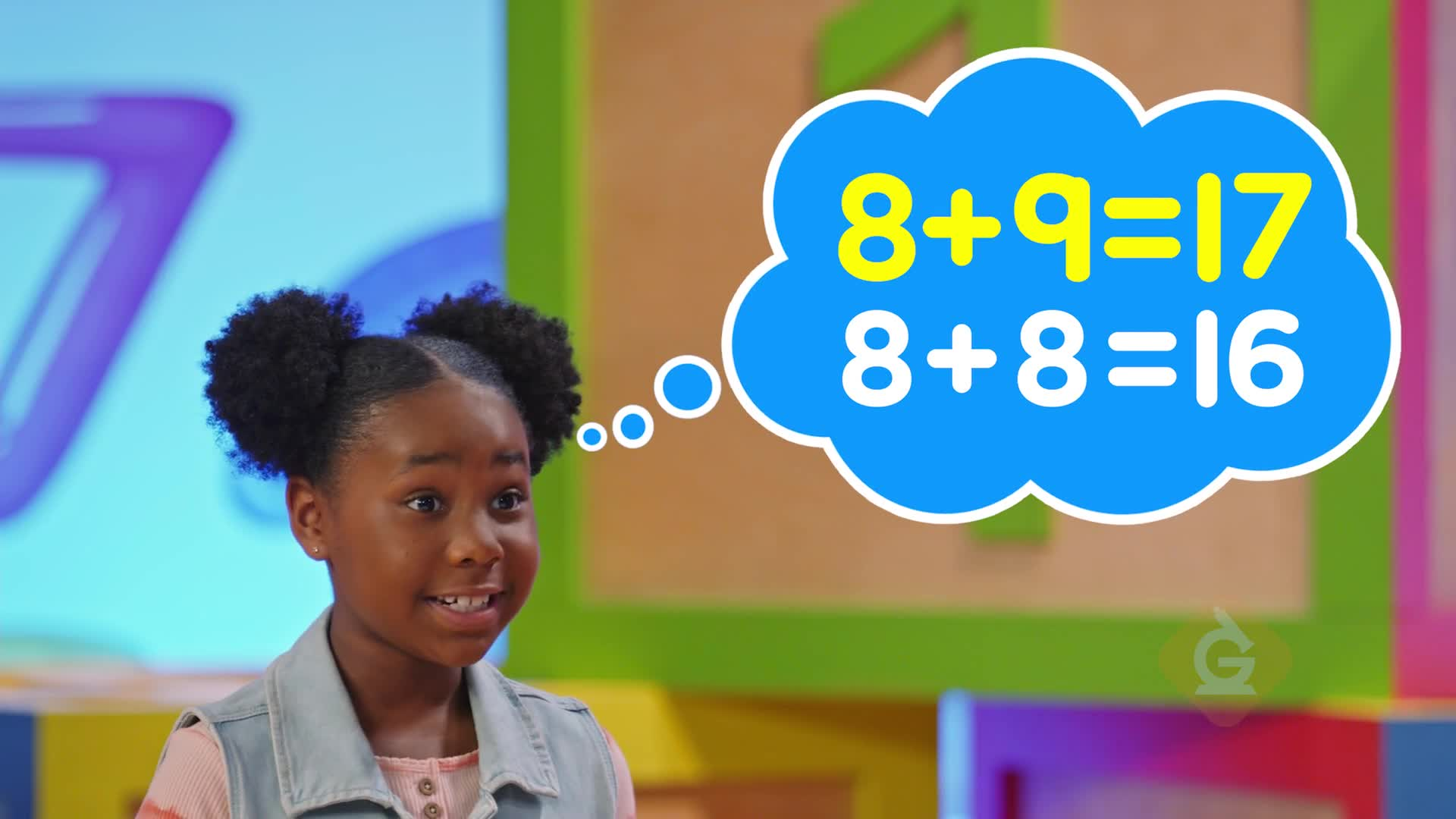WHAT IS MENTAL MATH WITHIN 100?

Mental math is math that you do without using a pencil and paper. Friendly numbers and skip counting can help you add and subtract using mental math.

To better understand mental math within 100…

WHAT IS MENTAL MATH WITHIN 100?. Mental math is math that you do without using a pencil and paper. Friendly numbers and skip counting can help you add and subtract using mental math. To better understand mental math within 100…

## LET’S BREAK IT DOWN!

### What are friendly numbers?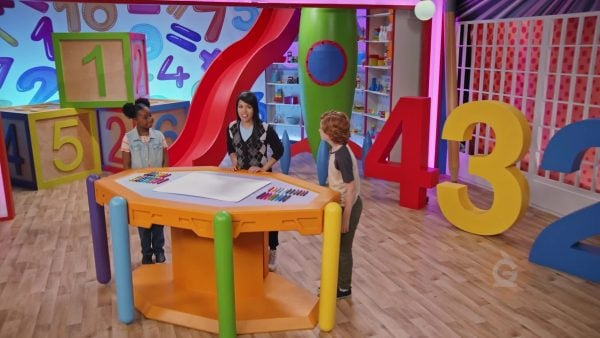Friendly numbers are numbers that are easy to add. It is easy to add 10 and another number. 10 + 2 = 12. It is easy to add together numbers that make a ten. 4 + 6 = 10. Doubles are also easier to add. 6 + 6 = 12.

What are friendly numbers? Friendly numbers are numbers that are easy to add. It is easy to add 10 and another number. 10 + 2 = 12. It is easy to add together numbers that make a ten. 4 + 6 = 10. Doubles are also easier to add. 6 + 6 = 12.

### Find how many crayons in all.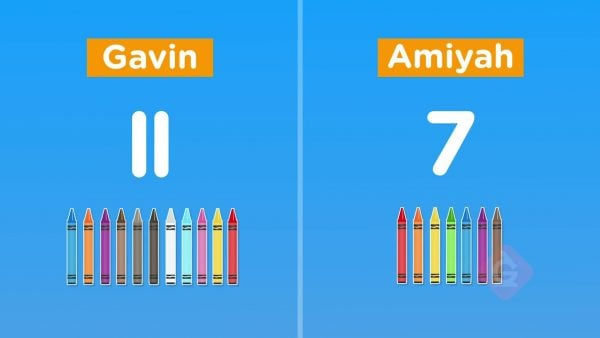You have 11 crayons. A friend has 7 crayons. How many crayons in all? 11 + 7 = ? To make friendlier numbers, take 1 away from 11 to get 10. Then move 1 to the 7 to get 8. 10 + 8 = 18. There are 18 markers in all.

Find how many crayons in all. You have 11 crayons. A friend has 7 crayons. How many crayons in all? 11 + 7 = ? To make friendlier numbers, take 1 away from 11 to get 10. Then move 1 to the 7 to get 8. 10 + 8 = 18. There are 18 markers in all.

### Find how many flowers in all.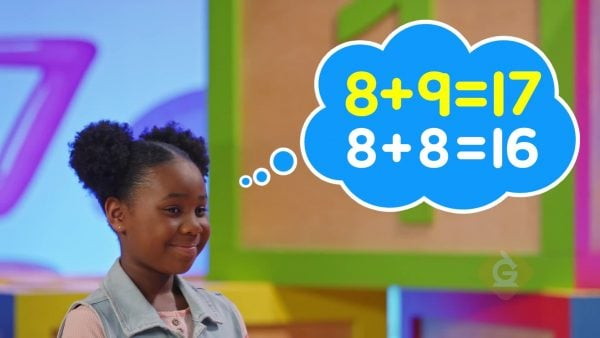You have 8 red flowers and 9 blue flowers. How many flowers in all? Use a double fact: 8 + 8 = 16. 8 + 9 is one more, so 8 + 9 = 17.

Find how many flowers in all. You have 8 red flowers and 9 blue flowers. How many flowers in all? Use a double fact: 8 + 8 = 16. 8 + 9 is one more, so 8 + 9 = 17.

### Find how many mice are left.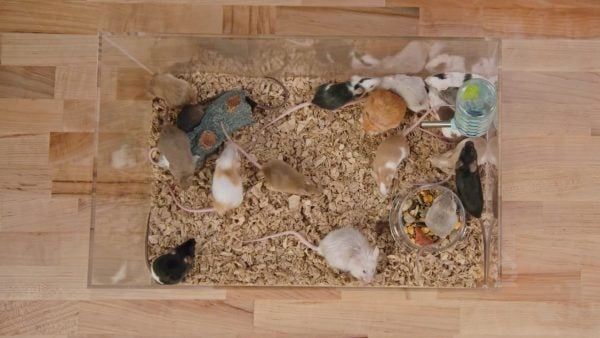There are 24 mice in a basket. 11 mice leave. How many are left? Find 24 – 11. It is easy to subtract 10. 11 is 10 and 1 more. 24 – 10 = 14. 14 – 1 = 13. There are 13 mice left.

Find how many mice are left. There are 24 mice in a basket. 11 mice leave. How many are left? Find 24 – 11. It is easy to subtract 10. 11 is 10 and 1 more. 24 – 10 = 14. 14 – 1 = 13. There are 13 mice left.

### Towers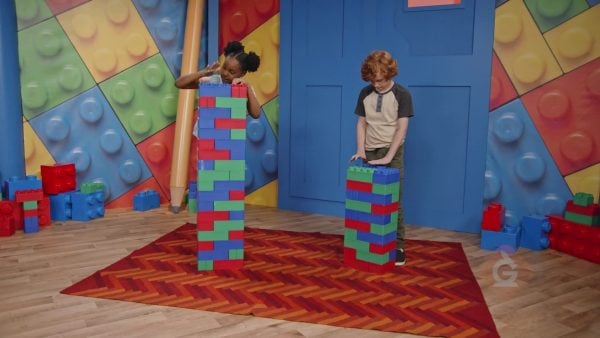There is a tower of 20 blocks. You add stacks of 10 blocks on top. To add groups of 10 you can skip count by 10. 20 + 10 = 30. To add 34 + 50, skip count by 10. Start at 34, then jump to 44, 54, 64, 74, 84. So, 34 + 50 = 84.

Towers There is a tower of 20 blocks. You add stacks of 10 blocks on top. To add groups of 10 you can skip count by 10. 20 + 10 = 30. To add 34 + 50, skip count by 10. Start at 34, then jump to 44, 54, 64, 74, 84. So, 34 + 50 = 84.

## MENTAL MATH WITHIN 100 VOCABULARY

To put amounts together.
Plus
The word used to describe adding two amounts.
Subtract
To find the difference between two amounts.
Minus
The word used to describe subtracting two amounts.
Mental Math
Solving problems without paper and pencil.
Friendly Numbers
Numbers that are familiar and work as easy starting points, like 10 and 20.
Counting by adding the same number over and over again.

## MENTAL MATH WITHIN 100 DISCUSSION QUESTIONS

### How can we use mental math to add 6 + 9?

We can subtract one from 6 and add one to 9. Then we have 5 + 10. We can also first add 4 to 6, so that we have 10, and then we can add the remaining 5, so we have 10 + 5 = 15. There is more than one way.

### Is there an easier way to subtract 20 – 17 than counting backward from 20?

Yes. We can count on from 17: 18, 19, 20. We counted 3 numbers, so 20 – 17 = 3.

### How can we use mental math to subtract 11 – 4?

We can first subtract 1 from 11 to make 10. Then subtract 3 more so we are subtracting 10 – 3, which is easier. We can also count on from 4 to 11.

### What happens to the tens place when we add 40 + 30? What happens to the ones place?

The tens place changes but the ones place stays the same.

### What happens to the tens place when we subtract 53 – 20? What happens to the ones place?

The tens place changes but the ones place stays the same.
X

## Success

We’ve sent you an email with instructions how to reset your password.
Ok

## Choose Your Free Trial Period### 7 DaysContinue to Lessons### 90 DaysGet 90 days free by inviting other teachers to try it too.

Share with Teachers

## Get 90 Days Free

### By inviting 4 other teachers to try it too.

4 required

Skip, I will use a 7 days free trial

## Thank You!

Enjoy your free 90 days trial